Here we are providing Class 11 chemistry Important Extra Questions and Answers Chapter 8 Redox Reactions. Chemistry Class 11 Important Questions are the best resource for students which helps in Class 11 board exams.

## Class 11 Chemistry Chapter 8 Important Extra Questions Redox Reactions

### Redox Reactions Important Extra Questions Very Short Answer Type

Question 1.
What are redox reactions? Give an example.
Redox reaction is a reaction in which oxidation and reduction take place simultaneously, e.g.
Zn (s) + Cu2+(aq) → Zn2+(aq) + Cu(s)

Question 2.
Define oxidation and reduction in terms of electrons.
Oxidation involves loss and reduction involves the gain of electrons.

Question 3.
Define an oxidizing agent. Name the best oxidizing agent.
The oxidizing agent is a substance that can gain electrons easily. F2 is the best oxidizing agent.

Question 4.
What is meant by reducing agent? Name the best reducing agent.
The reducing agent is a substance that can lose electrons easily. Li is the best reducing agent.

Question 5.
In the reaction MnO2 + 4HCl → MnCl2 + H2O which species is oxidized?
HCl is oxidized to Cl2

Question 6.
What is the oxidation state of Ni in Ni (CO)4?
Zero.

Question 7.
What is a redox couple?
The redox couple consists of the oxidized and reduced form of the same substance taking part in an oxidation or reduction half-reaction, for example.
Zn2+(aq) / Zn, Cl2 / Cl(aq) etc.

Question 8.
Define oxidation and reduction in the term of oxidation numbers.
Oxidation involves an increase in oxidation number while reduction involves a decrease in oxidation number.
Sn2+ + 2Hg2+ → Sn4+ + Hg2+

Here Sn2+ gets oxidised while Hg2+ gets reduced.

Question 9.
What is the sum of oxidation numbers of all atoms in HIO44?
Zero.

Question 10.
What is the oxidation number of N in (NH4)2 SO2?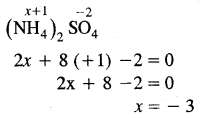The oxidation number of N is (NH4)2 SO4 is – 3.

Question 11.
What is the oxidation number of O in
(i) OF2
(ii) O2F2
The oxidation number of oxygen in OF2 is +2 whereas the oxidation number of oxygen in O2F2 is +1

Question 12.
At what concentration of M+(aq) will its electrode potential become equal to its standard electrode potential?
At the concentration of IMol / L.

Question 13.
Select the oxidant in the following reaction.
H2O2 + O3 → H2O + 2O2
O3 (zero to – 2)

Question 14.
What is the electrode potential of a standard hydrogen electrode?
Zero.

Question 15.
Set up an electrochemical cell for the redox reaction: Ni2+(aq) + Fe(s) → Ni(s) + Fe2+(aq)
Fe(s) / Fe2+(aq) || Ni2+(aq) / Ni(s)

Question 16.
What is the oxidation numbers of
(i) C in CH2O
Zero

(ii) pt in [pt(C2H4)Cl3]
2

Question 17.
What is the oxidation number of Mn in KMnO4?
The oxidation number of Mn in KMnO4 is
KMnO4 = 1 + x + 4 (- 2) = 0
x = + 7

Question 18.
What happens to the oxidation number of an element in oxidation?
It increases.

Question 19.
Which reaction occurs at the cathode in a galvanic cell?
Reduction.

Question 20.
Name one compound in which the oxidation number of Cl is +4.
ClO2.

Question 21.
If the reduction potential of an electrode is 1.28 v. What will be its oxidation potential?
– 1.28 v.

Question 22.
Can we store copper sulfate in an iron vessel?
No, because iron is more reactive than copper and thus holes will be developed in an iron vessel.
Cu2+ (aq) + Fe(s) → Fe2+(aq) + Cu(s)

Question 23.
The E° of Cu2+ / Cu is + 0.34 v. What does it signify?
Copper lies below hydrogen in the activity series.

Question 24.
Calculate the oxidation number of underlined elements in the followings:
Na2B4O7,O3O4
Na2B4O7 = 2 + 4x – 14 = 0
4x = 12
x = 3 .
O3O4 = x – 8 – 0
x = + 8

Question 25.
What is the oxidation number of alkali metals in its compounds?
+1.

Question 26.
Determine the change in the oxidation number of S in H2S and S02 in the following reaction:
2H2S(g) + SO2(g) → 3S(s) + 2H2S(g)
The oxidation number of S changes from – 2 in H2S and +4 in SO2 to zero in elemental sulfur.

Question 27.
Can we use KCl as an electrolyte in the following cell?
Cu(s) | Cu2+(aq) || Ag+(aq)| Ag(s)
KCl cannot be used as an electrolyte as a salt bridge because Cl ions will combine with Ag+ ions to form a precipitate of AgCl.

Question 28.
Define the EMF of the cell.
EMF of the cell is defined as the difference in the electrode potential of the two half cells when the cell is not sending current through the circuit.

Question 29.
A solution of Na2SO4 was electrolyzed by using some inert molecules. What are the products at the electrodes?
O2 & H2.

Question 30.
The reduction potentials are:
Cl2 + 2e → 2Cl – E° = 1.36V
F2 + 2e → 2F- E° = 2.87 V
Which is the better oxidising agent?
F.

Question 31.
What is the relationship between standard oxidation potential and standard reduction potential?
Both are equal in magnitude but opposite in sign.

Question 32.
Determine the oxidation number of nitrogen in HNO3.
HNO3
1 + x +. 3(- 2) = 0
x = + 5

Question 33.
What is the oxidation number erf Fe in Na4[Fe(CN)6]?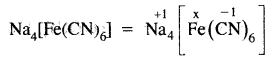x – 1 × 6 = – 4 ,
x = +2

Question 34.
What is the maximum and minimum oxidation number of Nitrogen?
The highest oxidation number of N is +5 and its minimum oxidation number is -3.

Question 35.
What is the basic principle of balancing the redox reactions by the ion-electron method?
A number of electrons lost during oxidation = number of electrons gained during reduction.

Question 36.
What is the oxidation state of S in H2SO4 & H2SO3?
H2SO4 = 2 ( + 1) + x + 4 (- 2) = 0
x = 8 – 2 = +6 ‘ ,
H2SO3 = 2 ( + 1) + x + 3 ( – 2) = 0
x = +4

Question 37.
What is the oxidation number of Xe in Ba2XeO2?
Ba2XeO2 = 2 × 2 + x + 2( – 2) = 0
4 + x – 4 = 0
x = 0

Question 38.
Define half cell.
The combination of an electrode and the solution in which it is dipped is called a half-cell.

Question 39.
Indicate the oxidizing and reducing agent in the following reaction:
2Cu2+ + 4I ⇌ 2CuI + I2
Cu2+ is an oxidizing agent and I is the reducing agent.

Question 40.
Write correctly the balanced half-reaction and the overall reaction for the following skeletal equation.
Fe(OH)2 + H2OZ ⇌ Fe(OH)3 + H2O (in basic medium)
[Fe(OH)2 + OH ⇌ Fe(OH)3 + e] × 2
[H2O2 + 2e ⇌ 2OH]
2Fe(OH)2 + H2O2 ⇌ 2Fe(OH)3

Question 41.
Calculate the oxidation number of P in MgP2O7
MgP2O7 = 2 × 2 + 2x + 7(-2) = 0
2x = 10
x = + 5

Question 42.
Arrange the following in order of increasing oxidation number of Mn:
MnCl2, MnO2, Mn(OH)3, KMnO4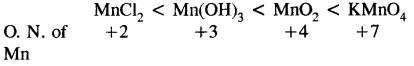Question 43.
Arrange the followings in order of decreasing the oxidation number of oxygen:
HXO4, HXO3, HXO2, HXOQuestion 44.
Arrange the following in order of increasing oxidation number of iodine:
I2, HI, HIO4, ICl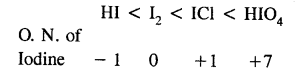Question 45.
How do you arrive at the oxidation number of Cr in Cr2O72-?
(Cr2O7 )2 = 2x – 14 = – 2
x = + 6

Question 46.
What is the charge on I mole of electrons?
96500 coulombs.

Question 47.
Set up an electrochemical cell for the redox reaction.
Ni2+(aq) + Fe(s) ⇌ Ni(s) + Fe2+(aq)
Fe(s) / Fe2+(aq) || Ni2+(aq) / Ni(s)

Question 48.
Define oxidation number?
The oxidation number of an element may be defined as the charge which an atom of the element has in its ions. It is also known as the oxidation state.

Question 49.
Define electrode potential.
The tendency, of an electrode to lose or gain electrons is called electrode potential.

Question 50.
Identify the strongest and weakest reducing agent from the metal.
Zn, Cu, Ag, Na, Sn
Strongest reducing agent = Na Weakest reducing agent = Ag

### Redox Reactions Important Extra Questions Short Answer Type

Question 1.
HNO3 acts only as an oxidant whereas HNOz acts both as an oxidant and reductant. Why?
Ox. No. of N in HNO3 = +5
Ox No. of N in HNO2 = +3
Maximum oxidation numbers which N can show is = + 5
(∴ It has only 5 valance electrons 2S22P3)

The Ox. No. of N in HNO3 is maximum and it can only decrease. Therefore HNO3 can act only as an oxidant. Minimum Ox. No. of N is -3.

Thus HNO2 in which Ox. No. of N is +3 Can decrease as well as increase. Thus HNO2 can act as an oxidant as well as a reductant.

Question 2.
Balance the following equation by the ion-electron method.
Zn(s) + NO3 → Zn2+(aq) + NH+ (aq) + H2O(l) (In acid solution)
Oxidation half reactionMultiply (1) equation by 4 to equalise the no. of electron in both. Add both half reaction
4 Zn(s) → Zn2+(aq) + 8e
Zn(s) + NO3 (aq) + 10H+ (aq) → 4Zn2+ (aq) +NH+(aq)+ 3H22O(1)
– 1 + 10 = + 8 + 1
+ 9 = + 9

Question 3.
Balance the following equation in acidic medium by oxidation number method.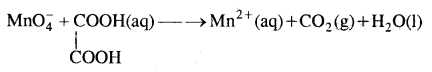Question 4.
Indicate the oxidising and reducing agent in the following reactions:
(i) 2Mg + SO2 → 2MgO + S
Mg = Reducing agent
SO2 = Oxidising agent

(ii)2Cu2+ + 41 → 2CuI + I2
Cu2+ = Oxidising agent
I- = Reducing agent

(iii)SO2 + 2H2S → 2H2O + 3S
SO2 = Oxidising ageiit
H2S = Reducing agent

(iv)Sn2+ + 2Hg2+ → Hg22+ + Sn4+
Sn2+ = Reducing agent
Hg2+ = Oxidising agent

Question 5.
Which of the following redox reaction is oxidation & which is reduction?
(i) Zn → Zn2+ + 2e
Oxidation
(ii) Cl2 + 2e → 2Cl-
Reduction
(iii) Fe → Fe2+ + 2e
Oxidation
(tv) Sn4+ + 2e → Sn2+
Reduction

Question 6.
What are the minimum and maximum oxidation numbers shown by sulfur?
The minimum oxidation number shown by S is – 2 since it can acquire 2 more electrons to achieve the nearest inert gas [Ar] configuration.

The maximum Ox. No. shown by S is +6 since it has 6 valance electrons. (3S2 3P4)

Question 7.
Arrange the molecule NH3, NO2, HN3, NO2 & N2H4 in the decreasing order of the oxidation state of nitrogen.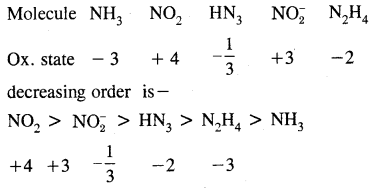Question 8.
Can the reaction.
Cr2O7 2- + H2O ⇌ 2CrO42- + 2H+ be regarded as redox reaction.
Ox. No. of Cr in Cr2O72- = + 6
Since the ox. no if Cr in CrO42- = + 6

There is no change in ox. no. in the reaction therefore this reaction cannot be regarded as a redox reaction.

Question 9.
Balance the oxidation—reduction reaction
FeS2 + O2 → Fe2O3 + SO2
Fe2+ → Fe3+ 1 ↑
S2-1 → 2S+4 10 ↑
O2 → 2O2- 4↓ × 11
4[Fe+2 + S2-1] + 11O2 → 4Fe3+ + 8S+4 + 22O-2
or
3FeS2 + 11O2 → 2Fe2O3 + 8SO2

Question 10.
Write the following redox reactions using half cell reactions:
(i) Zn(s) + FeCl2(aq) → ZnCl2(aq) + Fe(s)
(Zn(s) → Zn2+(aq) + 2e
Fe2+(aq) + 2e → Fe(s)

(ii) Mg(s) + Cl2(g) → MgCl2(s)
Mg(s) → Mg2+ + 2e
Cl2(g) + 2e → 2Cl (aq)

(iii) Mg(s) + 2H+(aq) → Mg2+(aq) + H2(g)
Mg(s) → Mg2+ + 2e
2H+(aq) + 2e → H2(g)

Question 11.
How would you know whether a redox reaction is taking place in an acidic/alkaline or neutral medium?
If H+ or any acid appears on either side of the chemical equation, the reactions take place in the acidic medium. If OH or any base appears on either side of a chemical equation, the solution is basic. If neither H+; OH nor any acid or base is present in the chemical equation, the solution is neutral.

Question 12.
Is it possible to store:
(i) Copper sulphate solution in a zinc vessel.
we cannot place CuSO4 solution in a zinc vessel, if the following redox reaction occur.
Zn + CuSO4 → ZnSO4 + Cu
or
Zn + Cu2+ → Zn2+ + Cu

By convention, the cell may be represented by
Zn / Zn2+ || Cu2+ / Cu
cell = E°cu2+ cu- E°zn2+
Zn = 0.34 -(- 0.76) = + 1.10v.
Since EMF comes out positive, therefore CuSO4 reacts with zinc. So CuSO4 solution cannot be stored in a zinc vessel.

(ii) Copper sulphate solution in silver vessel.
We cannot store CuSO4 solution in a silver vessel if the following reaction occurs.
2Ag + Cu2+ → 2Ag+ + Cu

By convention, the cell of the above redox reaction may be represented by
Ag / Ag+ || Cu2+ / Cu and E°cell = E°cu2+ , cu, E°Ag+
Ag = 0.34 – 0.80 = – 0.56 v.

Since the EMF of the cell is -ve, therefore CuSO4 does not react with silver or CuSO4 solution cannot be stored in a silver vessel.

Question 14.
The electrode potential of four metallic elements (A, B, C, D) are +0.80, -0.76, +0.12 and +0.34v. respectively. Arrange them in order of decreasing electron positive character.
Higher the electrode potential, (E°) lower is the tendency of the metal to lose electrons .and hence lower is the electropositive character. So the electrode potential increase in the order of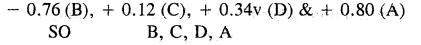Question 15.
What are the maximum and minimum oxidation numbers of N?
The highest ox. no. of N is +5 since it has five electrons in the valence shell (2S2 2P3) and its minimum o.no. is -3 since it can accept three more electrons to acquire the nearest inert gas (Ne) configuration.

Question 16.
Find out the ox. no. of Cl in HCl, HClO, ClO;, CaOCl2 & ClO2.
ox. no. of Cl = -1 in HCl, +1 in HClO, + 7 in ClO40, in CaOCl2 and + 4 in ClO2.

Question 17.
What will happen if a nickel spatula is used to stir a solution of copper sulfate?
Since E° of Ni (-0.25v) is lower than that of copper (+ 0.34v), therefore nickel has a higher tendency to lose electrons & copper has a higher tendency to gain electrons. Cu will deposit over the nickel spatula.

Question 18. Justify the reaction:
2Cu2O(S) + Cu2S (s) → 6Cu (s) + SO2 (g) is a redox reaction. Identify the species oxidized, reduced, which acts as an oxidant and which acts as reductant.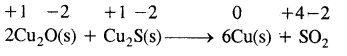In this reaction copper is reduced from + 1 oxidation state to zero oxidation State and sulfur is oxidized from – 2 to +4 state. Therefore the reaction is a redox reaction further Cu2O helps sulfur in Cu2S itself and Cu2O to decrease its oxidation number hence sulfur of Cu2S is a reducing agent.

Question 19.
Write two information about the reaction-
Mg + H2SO4 → MgSO4 + H2

1. Magnesium reacts with sulphuric acid to form magnesium sulfate and hydrogen.
2. 24 grams of magnesium reacts with 98 gm of sulphuric acid-producing (24 + 32 + 64) = 120 gm of magnesium sulfate and 2 gm of hydrogen.

Question 20.
Calculate the oxidation number of N in H – C = N
As nitrogen is more electronegative than carbon, therefore, each covalent bond contributes one unit negative value to the oxidation number to nitrogen. Thus o. no. of nitrogen in HCN = -3

Question 21.
I2 & Br2 are added to a solution containing Br- and I- ions. What reaction will occur if,
I2 + 2e → 21, E° = 0.54 v
& Br2 + 2e → 2Br, E° = + 1.09 v?
Since E° of Br2 is higher than that of I2, therefore Br2 has a higher tendency to accept electrons than I2. Conversely I- ions have a higher tendency to lose electrons than Br ions. Therefore, the following reaction will occur.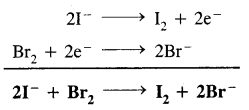In other words, I ions will be oxidized to I2 while Br2 will be reduced to Br ions.

Question 22.
Is it possible to store copper sulfate solution in a gold vessel?
We can store CuSO4 solution in a gold vessel if the following redox reaction occur
2Au + 3Cu2+ → 2Au3+ + 3Cu
The cell corresponding to the above redox reaction may be represented as:
Au / Au3+ || Cu2+ / Cu and E°cell = E°Cu2+ , Cu E°Au3+
Au = 0.34 – 1.50 = 1.16 v

since the EMF of the above cell reaction is – ve, therefore, CuSO4 solution does not react with gold in other words, the CuSO4 solution can be stored in a gold vessel.

Question 23.
When magnesium ribbon burns in air two products are formed, magnesium oxide and magnesium nitride point out the oxidizing and reducing agent.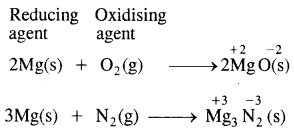Question 24.
What is the basic difference between electrochemical and electrolytic cells?
In electrochemical cells electrical energy is generated as a result of the redox reaction which occurs in an electrolytic cell, the chemical reaction takes place as a result of electrical energy supplied.

Question 25.
Why are articles made of iron coated with zinc to check their rusting?
Coating a layer of zinc on iron articles is called galvanization articles made up of iron are coated with zinc since zinc forms a protective coating on iron. When such articles are exposed to air. Zinc is oxidized to Zn2+ ions in preference to iron. Therefore zinc sacrifices itself for the sake of iron. The rusting of iron is prevented.

Question 26.
Identify the oxidizing agent, reducing agent, and the substance undergoing oxidation & reduction in the following reaction.
H2SO4 + 2HBr → SO2 + Br2 + 2H2O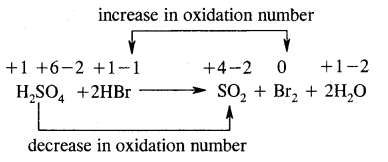Oxidizing agent – H2SO4
Reducing agent – HBr
Substance oxidized – HBr
Substance reduced – H2SO4

Question 27.
Consider the following galvanic cell.
Cd / Cd2+ (1M) || H+(1M) / H2(g / atm) pt
(i) Write the overall cell reaction
The anodic reaction is
Cd(s) → Cd2+(aq) + 2e

The Cathodic reaction is
2H+(aq) + 2e → H2(g)

The overall reaction is
Cd(s) + 2H+(aq) → Cd2+(aq) + H2(g)

(ii) What do the double vertical lines denote?
The double vertical lines denote the salt bridge which connects the oxidation & reduction half cells.

Question 28.
Write the following redox reaction in the oxidation & reduction half-reaction.
(a) 2K(s) + Cl2(g) → 2KCl(s)
K(s) → K+(aq) + e (Oxidation)
Cl2(g) + 2e → 2Cl (Reduction)

(b) 2Al(s) + 3Cu2+(aq) → 2Al3+(aq) + 3Cu(s)
Al(s) → Al3+ (aq) + 3e (Oxidation)
Cu2+ + 2e → Cu(s) (Reduction)

Question 29.
Find out the oxidation number of iron in
[Fe(H2O)5 (NO)+] SO4
[Fe(H2O)5(NO)+]2+
x + 0 + 1 = 2
x = 2 – 1
= +1

Question 30.
Calculate the oxidation number of all the atoms in- CrO42-.
If x is the ox. no. of Cr in CrO42- ion then
x + 4 × (- 2) x = – 2
x = 8 – 2 = 6
x = 6
For O → 6 + 4(x) = – 2
4x = – 8
x = $$\frac{-8}{4}$$ = – 2

### Redox Reactions Important Extra Questions Long Answer Type

Question 1.
Give the rules on the basis of which oxidation numbers are assigned to various elements.
Various atoms are assigned oxidation number on the basis of the following rules:

1. An element in the free state has an oxidation number equal to zero, e.g. H2, He, K, Ag all have zero ox. no.
2. In a binary compound of a metal and a non-metal, the oxidation number of metal is positive while that of non-metal is negative. In NaCl the ox. no. of sodium +1 and ox. n. of chlorine is  -1.
3. In a covalent compound, the atom with higher electronegativity has a negative oxidation number while another atom has a positive oxidation number.
4. The oxidation number of the radical or ions is equal to the electrical charge on it. for e.g. the ox. no. of Na+ is +1.
5. In neutral molecules, the algebraic sum of the oxidation number of all the atoms is zero.

Question 2.
Starting with the correctly balanced half-reaction, write the overall net ionic equation for the following change:
Chloride ion is oxidised to Cl2 by MnO4 (in acid solution)
The skeletal equation is
MnO4+ H+ + Cl(aq) → Mn2+ + Cl2(g) + H2O (l)

Ox. no. of Mn change from +7 in MnO4 to +2
Whereas ox. no. of chlorine change from -1 in Cl ions to 0 in Cl2.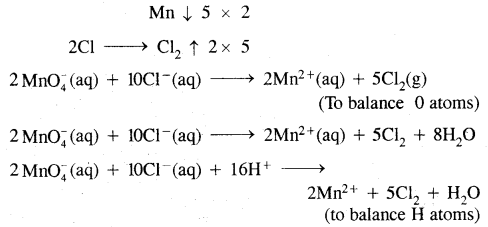Question 3.
Write the method used for balancing redox reaction by oxidation number method.
The following steps are used for balancing the reactions by this methods:

1. Writing the skeletal equation for all the reactants and products of the reaction.
2. Assignment of the oxidation number of all atoms in each compound in the skeletal equation. Identify the atoms undergoing a change in their oxidation number.
3. Calculating the increase or decrease in oxidation number per atom and then for the whole molecule in which it occurs. If these are not equal then multiplying by suitable coefficients such that these become equal.
4. Now balancing the chemical reaction with respect to all atoms except H & O.
5. Finally balancing with respect to H & O atom for balancing oxygen atoms add H20 molecules to the side deficient in it.

### Redox Reactions Important Extra Questions Numerical Problems

Question 1.
Determine the oxidation number of O in the following: OF2, Na2O2 & CH3COOH
(i) OF2
Let the ox. no. of O = x
The ox. no. of each F = — 1
x – 2 = 0
x = +2

(ii) Na2O2
Let the o. no. of O = x
ox. no. of each Na = + 1
2 + 2x = 0
2x = – 2
x = – 1

(iii) CH3COOH
Let the ox. no. of O = x
The ox. no. of each carbon atom = — 1
The ox. no. of hydrogen = +1
– 2 + 4 + 2x = 0
2x + 2 = 0
x = – 1

Question 2.
Determine the volume 6f M/8 KMnO4 solution required to react completely with 25.0 cm3 of M/4 FeSO4 solution in an acidic medium.
The balanced ionic equation for the reaction is
MnO4 + 5Fe2+ + 8H+ → Mn2+ + 5Fe3+ + 4H2O
from the balanced equation, it is evident that-
1 mole of KMnO4 = 5 moles of FeSO4

Applying the molarity equation to the balanced redox equation.Thus the volume of M/8 KMnO4 solution required = 10.0 ml.

Question 3.
How many grams of K2Cr2O7 is required to oxidize Fe2+ present in 15.2 gm of FeSO4 to Fe3+ if the reaction is carried out in an acidic medium.
The balanced chemical equation for the redox reaction is
K2Cr2O7 + 6FeSO4 + 7H2SO4 → K2SO4 + Cr2(SO4)3 + 7H2O
from the balanced equation, it is clear that 6 moles of FeSO4 = 1

a mole of K2Cr2O7 or 6 × 152 gm of FeSO4 are oxidized by
K2Cr2O7 = 294 gm
or
15.2 gm of FeSO4 are oxidized by K2Cr2O7

Question 4.
15.0 cm3 of 0.12 M KMnO4 solution are required to oxidize 20 ml of FeSO4 solution in an acidic medium. What is the concentration of FeSO4 solution?
The balanced chemical equation for the redox reaction is
2KMnO4 + 10FeSO4 + 8H2SO4 → K2SO4 + 2MnSO4 + 5Fe2(SO4)3 + 8 H2O

Applying molarity equation to the above redox reaction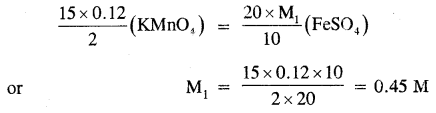Question 5.
16.6 gm of pure KI was dissolved in water and the solution was made up to one liter, cm3 of this solution was acidified with 20 cm3 of 2 MHCl the resulting solution required 10 cm3 of decinormal KIO3 for complete oxidation of I- ions to ICl. Find out the value of v.
The chemical equation for the redox reaction is
IO- + 2I + 6HCl → 3ICl + 3Cl + 3H2O

Molarity of KI solution = $$\frac{16.6}{166}$$ = 0.1 m

Applying molarity equation
$$\frac{0.1 \times v}{2}$$ (KI) = $$\frac{10 \times 0.1}{1}$$(KIO3)
v = 20 cm3

Question 6.
Calculate the cone of hypo (Na2S2O3 5H2O) solution in g dm-3 if 10.0 of this solution decolorized 15 ml of M/40 iodine solution.
The balanced equation for the redox reaction is
2S2O32- + I2 → 2I + S4O62-
from the balanced equation, it is evident that
2 moles of Na2S2O3 = 1 mole of I2

Applying the molarity equation we have,Thus, the molarity of the hypo solution = 3/40 M
mol. mass of Na2S2O3.5H2O = 248 g mol-1
cone, of Na2S2O3.5H2O = $$\frac{248 \times 3}{40}$$
= 18.6 gdm-3

Question 7.
How many millimoles of potassium dichromate is required to oxidize 24 cm3 of 0.5 M mohr’s salt solution in an acidic medium.
No. of millimoles of K2Cr2O7 present in 24 cm3 of 0.5 m solution = 24 × 0.5 = 12
The balanced chemical equation for the redox reaction is
K2Cr2O7 + 6(NH4)2SO4.FeSO4.6H2O + 7H2SO4 → K2SO4 + 6(NH4)2SO4 + 3Fe2(SO4)3 + Cr2(SO4)3 + 43H2O from the balanced equations.

6 moles mohr’s salt are oxidised by K2Cr2O7 = 1 moles
∴ 12 millimoles of mohr’s salt will be oxidised by
K2Cr2O7 = $$\frac{1}{6}$$ × 12 = 2 millimoies.

Question 8.
2.48 gm of Na2S2O3.xH2O was dissolved per liter of the solution. 20 cm3 of this solution required 10 cm3 of 0.01 M iodine solution. Find out the value of x?
The balanced equation for the redox reaction is
2Na2S2O3 + I2 → Na2S4O6 + 2NaI
Let the molarity of Na2S2O3 .xH2O solution = M1.

Applying molarity equation to the above redox reaction, we have
$$\frac{\mathrm{M}_{1} \times 20}{2}$$(Na2S2O3) = $$\frac{10 \times .01}{1}$$(I2)
∴ M1 = 0.01 M

mol wt. of Na2S2O3.xH2O
= 2 × 23 + 2 × 32 + 3 × 16 + x × 18
= 158 + 18x

Amount of Na2S2O3.xH2O present per litre
= (158 + 18x) × 0.01 g

But the actual amount dissolved = 2.48 g. equating these values, we have
(158 + 18x) × 0.01 = 2.48
or
x = 5.

Question 9.
The half cell reactions with their oxidation potentials are:
Pb(s) → Pb2+(aq) + 2e, E°oxi = + 0.13 v.
Ag(s) → Ag+(aq) + e, E°oxi = – 0.80 v.
Write the cell reaction and calculate its EMF.
The equations are as
Pb2+(aq) + 2e → Pb(s), E°oxi = – 0.13 v
Ag+(aq) + e → Ag(s), (E° = + 0.80 v) …(2)

To obtain the equation for the cell reaction, multiply equation (2) by 2 and subtract equation (1) from it we get
Pb(s) + 2Ag+(aq) → Pb2+(aq) + 2Ag(s)
cell = + 0.80 – ( – 0.13) = + 0.93 v

Question 10.
Predict whether zinc or silver reacts with 1 M H2SO4 to give out hydrogen or not. Given that the standard potential of zinc & silver are – 0.76 v & + 0.80 v respectively.
(a) To predict the reaction of zinc with H2SO4 If Zn reacts, the following reactions should take place.
Zn + H2SO4 → ZnSO4 + H2
i.e. Zn + 2H+ → Zn2+ + H2

By conventions, the cell will be represented as-
Zn / Zn2+ ∥ H+ / H2

standard EMF of the cell,
E°cell = E°H+/H2 – E°zn2+/zn
= 0 – (- 0.76) = + 0.76 v

Thus the EMF of the cells comes out to be positive. Hence the reaction takes place.

(b) To predict the reaction, of silver with H2SO4.
If Ag reacts, the following reactions should take place.
2Ag + H2SO4 → Ag2SO4 + H2
2Ag + 2H+ → 2Ag+ + H2

By convention, the cell may be written as
Ag / Ag+ ∥ H+ / H2
cell = E°H+,H2 – E°Ag+,Ag
= 0 – 0.80 = – 0.80 v.
The EMF of the cell is negative
Hence, this reaction does not take place.

Question 11.
Calculate the oxidation number of Sb atoms in Sb2O5
Sb2O5: Oxygen in common oxide has an oxidation state of -2. Therefore if x is the oxidation number of Sb in Sb2O5 then.
2 × x × 5 × (-2) = 0
x = $$\frac{10}{2}$$ = 5
The ox. no. of Sb in Sb2O5 = +5

Question 12.
Calculate the ox. no. of sulphur is Na2S2O3
Ox. no. of various atom in Na2S2O3
Na = +1, S = x, 0 = – 2
2( + l) + 2x + 3(- 2) = 0
2 + 2x – 6 = 0
2x = 4
x = + 2

Question 13.
Determine the oxidation number of O. in CH3COOH.
Let the ox. no. of O = x
The oxidation no. of each carbon atom = – 1
– 2 + 4 + 2x = 0
2x + 2 = 0
x = – 1

Question 14.
Determine the ox. no. of all the atoms in KClO4.
The ox.no. of K = +1
The ox. no. of Cl = x
The ox. no. of O = — 2
1 + x – 8 = 0
x – 7 = 0 .
x = 7
(K = +1, O = -2, Cl = 7)

Question 15.
Calculate the ox. no. of metal atom in Fe((CN)6)3-
Fe((CN)6)3-
The ox. no. of CN- = – 1

Let the ox. no. of Fe be x
x + 6 (- 1) = – 3
x = + 3

Question 16.
Find out the ox. no. of iron in
[Fe(H2O)5(NO)+]SO4
[Fe(H2O)5(NO)+]2+
x + 0 + 1 = 2
x = 2 – 1 = +1

Question 17.
Find out the ox. no. of chlorine in HCl & HClO.
(i) Cl in HCl is
+1 + x = 0
x = – 1

(ii) Cl in HCIO is
+ 1 + x – 2 = 0
x = +1

Question 18.
Find the ox. state of S in S2O42- or HSO3
(i) S2O42-
2x – 8 = – 2
2x = 6
x = +3

(ii) HSO3
+ l + x – 6 = – 1
x – 5 = – 1
x = + 4

Question 19.
Calculate the emf of the cell.
M1 = 1.00 M, M2 = 0.40 M, PH2 = 1.00 atm
[Pb2+(aq)]
LOOM (H+ (aq)) = 0.40 M
emf 0.00 – (- 0.13) + $$\frac{0.059}{2}$$ log $$\left(\frac{0.40}{1.00}\right)^{2}$$
= 0.13 – 0.0295 × 0.7959
= 0.13 – 0.023 = 0.107 v

Question 20.
Calculate the number of coulombs required to deposit 40.5g of aluminum when the electrode potential is
The atomic mass of Al is 27.
The number of coulombs required to deposit 27g of Al is
or
3 × 96500
Thus, the charge required to deposit 27 g of Al
= 3 × 96500 coulombs

Charge required to deposit 40.5 g of Al
= 3 × $$\frac{96500}{27}$$ × 40.5 = 434250 c.

Question 21.
Calculate the ox. number of oxygen in OF2
OF2
x – 2 = 0
x = +2

Question 22.
Calculate the ox. no. of Pb in Pb3O4
3x + 4( -2) = 0
3x = 8; x = $$\frac{8}{3}$$

Question 23.
Calculate the ox. no. of S in H2SO4.
H = +1, S = x, O = – 2
2 × 1 + x + 4 (- 2) = 0
2 + x – 8 = 0
x = + 6

Question 24.
Calculate the ox. no. of carbon atom in C6H12O6